# Does mesh analysis give no solution?

#### WBahn

Joined Mar 31, 2012
28,185
Hello again,

Here is a proof that a mesh current is not real. A current can not be two values at the same time.

View attachment 274923
NO ONE has ever claimed that mesh currents are anything other than a mathematical equivalent used as a tool for the purpose of simplifying circuit analysis. In some branches of some circuits (namely the outer edges), the physical currents happen to be the same as the mesh currents. But, in general, the physical currents are the differences of two mesh currents.

That is EXACTLY what the case is in this circuit. The CCVS is controlled by a physical current that is merely expressed as the difference of two mesh currents. The CCVS does not ever have to be aware of either mesh current individually, it is controlled by the difference of two mesh currents which is ALWAYS, by definition, equal to the physically-sensible current that controls it.

#### MrAl

Joined Jun 17, 2014
9,781
NO ONE has ever claimed that mesh currents are anything other than a mathematical equivalent used as a tool for the purpose of simplifying circuit analysis. In some branches of some circuits (namely the outer edges), the physical currents happen to be the same as the mesh currents. But, in general, the physical currents are the differences of two mesh currents.

That is EXACTLY what the case is in this circuit. The CCVS is controlled by a physical current that is merely expressed as the difference of two mesh currents. The CCVS does not ever have to be aware of either mesh current individually, it is controlled by the difference of two mesh currents which is ALWAYS, by definition, equal to the physically-sensible current that controls it.
So it sounds like you are telling me to put a shunt in series with Iin to measure the "mesh" current, which you still think we can measure just because it's outside of the loop that also encloses R1.
Is that correct?

#### MrAl

Joined Jun 17, 2014
9,781
No, the Xenon02 professor said that Iin = Is when we shorted out R2.
So the circuit in question does not contain R2 resistor.
View attachment 274941
And the task was to find Is current (p = R1).
Hello again Jony,

Well here is what i hear you telling me:
"There are two currents that are equal when we short out R2, but the circuit does not contain R2".
So which is it?It sounds like on the one hand we have R2 and just because it is shorted out we have a different circuit.
That makes some sense, but you can always put it back in and then let it tend to zero.
Once you put it back in you have the last solution i posted, then you can let it tend to zero.

It seems true that when the voltage is zero there is no current flow through R1 and thus all the input current has to go somewhere else, and that somewhere else is being called "Is".

Are you now saying that we MUST set p equal to R1?

#### MrAl

Joined Jun 17, 2014
9,781
Yup my proffesor said it is Iin = Is
Here are his calculations :

View attachment 274948
R1 = r
Thanks for the post but i cant read it. It is far too sloppy. See if you can make it more legible.

#### WBahn

Joined Mar 31, 2012
28,185
So it sounds like you are telling me to put a shunt in series with Iin to measure the "mesh" current, which you still think we can measure just because it's outside of the loop that also encloses R1.
Is that correct?
How many times can I say this? That CCVS does not EVER, EVER, EVER need to know or measure ANY mesh current! EVER!

It is controlled by the quantity (Ix-Ia) (using the notations on the TS's second diagram). It is NOT controlled by Ix. It is NOT controlled by Ia. It is controlled by the quantity (Ix-Ia). The quantity (Ix-Ia) IS the current I1, which IS the current flowing up through the R1 resistor, which IS the current that is controlling the CCVS in the original problem.

That these are mathematically equivalent is due to the circuit being linear. In linear circuits, superposition holds. Mesh current analysis is only valid for linear circuits. This is why it is only valid for linear circuits. But it IS valid for linear circuits and this IS a linear circuit.

#### dcbingaman

Joined Jun 30, 2021
855
I didn't stop here. This equation is not dimensionally consistent. You are saying that a voltage is equal to a pure number multiplied by a current. This is the exact same thing as saying that some objects weight is equal to some pure number multiplied by its height. It's a meaningless statement.

The problem is that you are CHANGING the equation based on what you WANT it to be.

Again, this is NO different then me giving you the equation

w = 1*h

and then telling you that I am 6 ft tall and you then using that equation to determine how much I weigh. You won't do so, no matter how many times I ask, because you know that this is a meaningless equation. The bottom line is that you can't guess what the units on that 1 are supposed to be, and so you dodge the question but you THINK you know what the units on the 1 in the other equation somehow "must" be and so you are okay with it.

It IS wrong. V and i are both symbolic quantities and thus carry their own units. V has units of energy per units charge and i has units of charge per unit time. But 1 is not a symbolic parameter, it is a specific quantity and quantities have both magnitude and units. You are claiming that the units on this particular quantity are dimensionless, when they aren't. They need to have dimensions of energy-time per charge. Ohm's is just one of many possible units that have that dimension.

Consider the distance-rate-time formula:

d = r*t

Each of those is a symbolic parameter and they each carry their own dimensions. The 'd' has the dimension of length, the 'r' has dimensions of distance per unit time, and the 't' has units of time.

Now let's say that I constrain 'r' to be a particular speed whose magnitude is 60. You are claiming that that should be written as

d = 60*t

Okay, so if t is then 2 minutes, how many feet has the object moved?

Are you going to claim that the units on that 60 must be feet per minute and then claim that the object moved 120 feet?

But what if, instead, I wrote that equation correctly as

d = (60 mph)*t

and then ask you the same exact question: If t is then 2 minutes, how many feet has the object moved?

Do you see that the '60' needs to have units? If they are left out, the equation is dimensionally inconsistent because the notion that distance is somehow equation to time multiplied by a pure number is meaningless.

Do you also see that not only is it not necessary, but it is wrong to say something like

d(feet) = (60 mph)*t(minutes)

What if 't' is in weeks, or seconds, or days? Why can't 'd' be in inches, or meters, light-seconds?

The following is perfectly correct:

10,560 ft = 60 mph * 2 minutes

That is every bit as correct as equation such as

72 inches = 6 feet

The gain of a current-controlled voltage source is a transimpedance. For some amount of current measured at the control point, the source produces a certain voltage out someplace else (hence the 'trans' in the name). The units therefore have dimensions of voltage per unit current. They might be volts/amp or mV/kA or kV/mA. It would be very natural to express the transimpedance as something like 1 V/A, but 1 V/A is also known as 1 Ω.

In a very real sense, a resistor is a current-controlled voltage source. Pass a current through it and it produces an output voltage. A 10 kΩ resistor produces 10 V/mA, or 10,000 V/A, or 10 mV/uA. A resistor, however, is not a 'trans'-impedance device, but rather just an impedance device.
Using dimensional analysis is very effective in determining if the math makes sense for just about any problem in physics/math/science. When solving difficult technical problems with complex algebra you can always check your answer for sanity by looking at the final units. If there is a mismatch then there is a mistake somewhere. Being human I am prone to mistakes when performing complex algebra. Many times I have caught mistakes by just verifying the final equality has dimensional equality.

#### MrAl

Joined Jun 17, 2014
9,781
How many times can I say this? That CCVS does not EVER, EVER, EVER need to know or measure ANY mesh current! EVER!

It is controlled by the quantity (Ix-Ia) (using the notations on the TS's second diagram). It is NOT controlled by Ix. It is NOT controlled by Ia. It is controlled by the quantity (Ix-Ia). The quantity (Ix-Ia) IS the current I1, which IS the current flowing up through the R1 resistor, which IS the current that is controlling the CCVS in the original problem.

That these are mathematically equivalent is due to the circuit being linear. In linear circuits, superposition holds. Mesh current analysis is only valid for linear circuits. This is why it is only valid for linear circuits. But it IS valid for linear circuits and this IS a linear circuit.
Sorry but you must not be reading my posts. I already agreed that Ix-Ia or Ia-Ix is a real current and therefore could be used to control a controlled source. If you looked at the post that proves that Ia is not real, you would have seen that.

The new question was about measuring Ia, not whether or not it could be used for anything. You said you can measure it, but you seem to fail to do that so far. Can you measure it or not? If you really can i would really like to see how.
Personally i dont see how you could measure something that does not exist, but if you can i would hope to see the method.

It does not matter for the math about the circuit, but if you state that you can measure it then measure it.
We all know that it works for mesh analysis, no question there. The question is can you measure it and if so how.
So it is not a question about using it for general circuit analysis. It's more specific. It's about the current Ia and Ia only.

Since you really seem to know a lot about this it would be very good to see how you measure Ia, and if you can, show how to measure Ix also.

#### dcbingaman

Joined Jun 30, 2021
855
Hello again,

I think for the subject of 'units' we are talking different contexts that's all it is. In some contexts we would have to be more explicit but if someone doesnt know that you can take that '1' to be similar to a gain then they are reading out of context. In this thread maybe you make a good point but i dont really want to debate that particular issue anymore because i know you are very strict about your units and apparently the context means nothing to you except for the purpose of 'correcting' them.
But see here's the thing ... if you knew how to 'correct' the units oringinally used then you must have been able to interpret the units BEFORE the correction, otherwise you could never suggest a correction because you wouldnt know what the "real" units were. In other words, you saw V=1*i and say that's wrong, but you were able to specify the units. How could you specify units if you didnt know what they were by seeing V=1*i . The only answer is that you could read them by the context, which is the circuit as well as the expression, not just the expression alone. When you isolate one thing by itself out of a massive amount of material that means you may be taking something out of context.
I wont say you are wrong though because i have seen papers written where the context is not clear. In those cases it may be much harder to interpret what units are being used. Some units are more important too such s distance, but i think volts are volts around the world, i could be wrong though.
But i dont think that is the main issue anyway. The main issue is the second drawing where a mesh current is being used as PART of a controlled source. We can talk about units anytime. So let me digress now by going back to the issue of a mesh current, real or not, measurable or not.

For your last paragraph, you would need to sense Ia if you put Ia into the expression for the voltage source.
If you put I1 into the expression then you can sense I1.

It's a known fact that you can not measure Ia, and because Ix is also a mesh current, you can not measure that either.

If you disagree with Ix being non measurable, then simply show me where you put the current shunt to measure that. if you cant do that, show me where you can put two current shunts to measure Ix, and if you cant do that, show me where you could put three current shunts to measure Ix, etc., etc.
If you rather stick to 'measuring' Ia, then do the same for that, use any number of current shunts you wish.
If you do not wish to draw the diagram with the shunt(s) then just tell me what line(s) you would like to see current shunts in to measure either Ia or Ix, but you'll have to show the polarity of the shunt(s). For example, if you put a shunt in series with Iin (perfectly reasonable btw) and you have the positive end of the shunt connected to the current source Iin, then the output voltage from the shunt will be positive at the end that connects to the top of the current source Iin.

"Again, it doesn't matter whether you can separately sense Ix and Ia, because the CCVS never has to do that. But, in this circuit, if you really, really, really felt the need, then put a shunt in series with the current source to sense Ia and put a shunt in series with R2 and then use a diff amp to take the difference. But why would you do that. Just measure the current in R1, is IS the quantity (Ix-Ia) that is controlling the source."

you seem to be implying that we put one current shunt in series with Iin such that the positive output would be taken at the top of Iin, and so anything that Iin is the shunt measures, then a shunt in series with R2 which i am not sure what polarity you would use, but lets say you put the positive end at the top of R1, then subtract.
If that's true (and i think i have the polarities right), then what you are measuring is, again, NOT Ia you would be measuring either I1 or -I1 (original, first diagram) because Iin minus iR2 equals I1 (or -I1 depending on polarities).
What that means is if you substituted this into the expression for V where Ia goes you get:
V=(Ix-I1)*p or you get V=(Ix+I1)*p
which would not be the right control value. The right value is V=I1*p with the polarity shown in the first diagram or V=-I1*p if you use the opposite polarity to measure I1.

So what this leads to is the statement that, you can never draw a schematic that shows a way to measure Ia (or Ix).
Are there some circuits that are contrary to that? There could be some symmetry that in some circuit it works, but i dont think so, and certainly not for this circuit, but you can try maybe you can come up with something. Personally i think it would be cool if you did.

Last but not least, the solution for vR1 for the original circuit using I1 as the control value is:
vR1=(Iin*R1*R2)/(R2+R1-p)

and those are all original values.
I guess we might want to specify that R1+R2 can not equal p unless maybe we allow for an infinite voltage at the top of R1. I dont think a matrix method would work then though.
I agree that the units might have been 'understood' when it comes to this simple example. But I have to agree that we need to specify units for constant scalar quantities. When we don't we are literally just 'inviting' trouble and causing confusion.

V = (1 V/A) * i

If I have this:

V = 1 * i

I can just drop the 1:

V = i

Which is a silly statement, but when I have this:

V = (1 V/A) * i

I cannot simply divide through by 1, I could divide through by 1V/A for:

V*(A/V)=i

which is valid, because the units prohibit me from dividing through without considering the very important units. V drops out leaving A:
A=i

Which is dimensionally correct.

#### WBahn

Joined Mar 31, 2012
28,185
Using dimensional analysis is very effective in determining if the math makes sense for just about any problem in physics/math/science. When solving difficult technical problems with complex algebra you can always check your answer for sanity by looking at the final units. If there is a mismatch then there is a mistake somewhere. Being human I am prone to mistakes when performing complex algebra. Many times I have caught mistakes by just verifying the final equality has dimensional equality.
And what I'm recommending goes a bit beyond that. Don't just check the final equality -- check EVERY step in the work. It seems arduous at first, but it doesn't take long before is it second nature. You largely no longer even realize you are doing it until some term in some step doesn't track properly. Then it catches you attention immediately (most of the time) and you can identify and fix the problem right there. In the end, it saves a HUGE amount of time because it prevents you from spending pages and hours on work that was guaranteed to be useless almost immediately.

But this only works if the units are tracked properly from beginning to end. This frequently means adding units to the initial problem because the person that presented the problem, be it a textbook author, an instructor, a customer, or another engineer in your same organization, was too lazy to do it properly.

Just the other day I was working a problem for another thread involving nonlinear components and thought I had set everything up correctly, but at the second line in my work the units didn't work out. I stopped and focused on why the units weren't working out and realized after a minute or so that I had misinterpreted one of the given relations, thinking it gave resistance as a function of current and not voltage as a function of current. A quick head slap and then on to the correct answer. If I had just blindly cranked numbers and tacked on the units I would have believed/wanted the answer to have, I would have had a result that was not at all unreasonable, but would have been completely wrong. Since it was a nonlinear circuit, no red flags would have gone off.

Tracking units is arguably the single most powerful error detection tool available to the engineer. Engineers that refuse to use it are, in my opinion, being grossly and potentially criminally negligent. Juries have agreed more than once after damages, injuries, or deaths have resulted.

•dcbingaman

#### dcbingaman

Joined Jun 30, 2021
855
And what I'm recommending goes a bit beyond that. Don't just check the final equality -- check EVERY step in the work. It seems arduous at first, but it doesn't take long before is it second nature. You largely no longer even realize you are doing it until some term in some step doesn't track properly. Then it catches you attention immediately (most of the time) and you can identify and fix the problem right there. In the end, it saves a HUGE amount of time because it prevents you from spending pages and hours on work that was guaranteed to be useless almost immediately.

But this only works if the units are tracked properly from beginning to end. This frequently means adding units to the initial problem because the person that presented the problem, be it a textbook author, an instructor, a customer, or another engineer in your same organization, was too lazy to do it properly.

Just the other day I was working a problem for another thread involving nonlinear components and thought I had set everything up correctly, but at the second line in my work the units didn't work out. I stopped and focused on why the units weren't working out and realized after a minute or so that I had misinterpreted one of the given relations, thinking it gave resistance as a function of current and not voltage as a function of current. A quick head slap and then on to the correct answer. If I had just blindly cranked numbers and tacked on the units I would have believed/wanted the answer to have, I would have had a result that was not at all unreasonable, but would have been completely wrong. Since it was a nonlinear circuit, no red flags would have gone off.

Tracking units is arguably the single most powerful error detection tool available to the engineer. Engineers that refuse to use it are, in my opinion, being grossly and potentially criminally negligent. Juries have agreed more than once after damages, injuries, or deaths have resulted.
True; It is definitely better to track your units at every step of the process. I think I kind of intuitively do that, but I should be more consistent as there is no doubt it reduces mistakes and errors.

#### dcbingaman

Joined Jun 30, 2021
855
And what I'm recommending goes a bit beyond that. Don't just check the final equality -- check EVERY step in the work. It seems arduous at first, but it doesn't take long before is it second nature. You largely no longer even realize you are doing it until some term in some step doesn't track properly. Then it catches you attention immediately (most of the time) and you can identify and fix the problem right there. In the end, it saves a HUGE amount of time because it prevents you from spending pages and hours on work that was guaranteed to be useless almost immediately.

But this only works if the units are tracked properly from beginning to end. This frequently means adding units to the initial problem because the person that presented the problem, be it a textbook author, an instructor, a customer, or another engineer in your same organization, was too lazy to do it properly.

Just the other day I was working a problem for another thread involving nonlinear components and thought I had set everything up correctly, but at the second line in my work the units didn't work out. I stopped and focused on why the units weren't working out and realized after a minute or so that I had misinterpreted one of the given relations, thinking it gave resistance as a function of current and not voltage as a function of current. A quick head slap and then on to the correct answer. If I had just blindly cranked numbers and tacked on the units I would have believed/wanted the answer to have, I would have had a result that was not at all unreasonable, but would have been completely wrong. Since it was a nonlinear circuit, no red flags would have gone off.

Tracking units is arguably the single most powerful error detection tool available to the engineer. Engineers that refuse to use it are, in my opinion, being grossly and potentially criminally negligent. Juries have agreed more than once after damages, injuries, or deaths have resulted.
I understand MrAI's perspective like in this: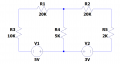We have two mesh currents that people normally write as follows and 'assume' the current is in milliamps:

This is by far less cumbersome to write: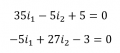Though technically that is this: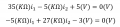Last edited:

#### WBahn

Joined Mar 31, 2012
28,185
Hello again,

Here is a proof that a mesh current is not real. A current can not be two values at the same time.

View attachment 274923
I2 is NOT the current flowing in R2. It is ONE COMPONENT of the current flowing in R2.

The current flowing in R2 is (I2-I3).

But I2 IS the current flowing in V1 and R1. Measure the current in R1 and, guess what, you have just measured I2.

Measure the current in R3 and, guess what, you have just measured I3.

Measure the current in R2 and, guess what, you have just measured (I2-I3).

•dcbingaman

#### WBahn

Joined Mar 31, 2012
28,185
I understand MrAI's perspective like in this:

View attachment 274991

We have two mesh currents that people normally write as follows and 'assume' the current is in milliamps:

This is by far less cumbersome to write:

View attachment 274992
I have little tolerance or sympathy for choosing to write things that are wrong just because doing so is less cumbersome.

I have seen many examples where someone does this because they assume it's obvious what the units are and then they make a mistake that tracking the units would have caught, but they don't catch it because they were too lazy to track their units. The consequences have varied all over the place, ranging from a few hours lost time, to a chip that cost $50k to fab and was worthless, to equipment being damaged, to someone dying a rather painful death and leaving a pregnant wife and a couple kids behind. I certainly can't stop people from being lazy and irresponsible with their units. But by the same token, I don't have to condone it or accept the "other people are sloppy and so that justifies me being sloppy" claims, either. Though technically that is this: View attachment 274993 But you should get out of the habit of putting the units in parens separate from the magnitude. The concrete value has BOTH a magnitude and a value. It is better to write it as (25 kΩ)*i1 - (5 kΩ)*i2 + 5 V = 0 Notice that when we have zero, we actually do not need to include units because zero is zero is zero. Now, there's a caveat and that is when we are dealing with a relative measurement scale (a.k.a, an affine scale) instead of an absolute scale (a.k.a., a linear scale). For example, the width of something is an absolute measurement, so if I say that the width of something is zero, it doesn't matter whether it is zero nanometers or zero lightyears, it is zero. But temperature in either °C or °F are relative measurements, and so zero on either of these scales HAS to have the units. Last edited: •dcbingaman #### dcbingaman Joined Jun 30, 2021 855 But you should get out of the habit of putting the units in parens separate from the magnitude. The concrete value has BOTH a magnitude and a value. It is better to write it as (25 kΩ)*i1 - (5 kΩ)*i2 + 5 V = 0 Notice that when we have zero, we actually do not need to include units because zero is zero is zero. Now, there's a caveat and that is when we are dealing with a relative measurement scale (a.k.a, an affine scale) instead of an absolute scale (a.k.a., a linear scale). For example, the width of something is an absolute measurement, so if I say that the width of something is zero, it doesn't matter whether it is zero nanometers or zero lightyears, it is zero. But temperature in either °C or °F are relative measurements, and so zero on either of these scales HAS to have the units. Agree, keeping the units with the magnitude is a better choice. #### dcbingaman Joined Jun 30, 2021 855 I have little tolerance or sympathy for choosing to write things that are wrong just because doing so is less cumbersome. I have seen many examples where someone does this because they assume it's obvious what the units are and then they make a mistake that tracking the units would have caught, but they don't catch it because they were too lazy to track their units. The consequences have varied all over the place, ranging from a few hours lost time, to a chip that cost$50k to fab and was worthless, to equipment being damaged, to someone dying a rather painful death and leaving a pregnant wife and a couple kids behind.

I certainly can't stop people from being lazy and irresponsible with their units. But by the same token, I don't have to condone it or accept the "other people are sloppy and so that justifies me being sloppy" claims, either.

But you should get out of the habit of putting the units in parens separate from the magnitude. The concrete value has BOTH a magnitude and a value. It is better to write it as

(25 kΩ)*i1 - (5 kΩ)*i2 + 5 V = 0

Notice that when we have zero, we actually do not need to include units because zero is zero is zero. Now, there's a caveat and that is when we are dealing with a relative measurement scale (a.k.a, an affine scale) instead of an absolute scale (a.k.a., a linear scale).

For example, the width of something is an absolute measurement, so if I say that the width of something is zero, it doesn't matter whether it is zero nanometers or zero lightyears, it is zero.

But temperature in either °C or °F are relative measurements, and so zero on either of these scales HAS to have the units.
I agree with you, though most textbooks I have read tend to write the above equations even more abbreviated as in: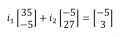or even more concise and drop the i1 and i2 (assume them):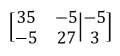Not that it is correct, but it happens a lot.

#### MrAl

Joined Jun 17, 2014
9,781
I2 is NOT the current flowing in R2. It is ONE COMPONENT of the current flowing in R2.

The current flowing in R2 is (I2-I3).

But I2 IS the current flowing in V1 and R1. Measure the current in R1 and, guess what, you have just measured I2.

Measure the current in R3 and, guess what, you have just measured I3.

Measure the current in R2 and, guess what, you have just measured (I2-I3).
Hello again,

You just wrote:
"But I2 IS the current flowing in V1 and R1. Measure the current in R1 and, guess what, you have just measured I2."

Very sorry but that is the same mistake that other people make too. They see the current loop arrow and follow it around then assume that it is the current in the wire just because they are used to seeing real current arrows that show the direction of the real current. I2 does not show anything real it is completely fictitious and can not be measured as i will show in this next diagram. The previous diagram had shown this too but the presence of the zero current in R2 may trip people up so i made the same circuit but with an imbalance so we would get three real currents to work with.
Notice that the currents shown with the small blue arrows next to the resistors are all real currents and every one of them can be measured.

Now looking at that new diagram, we see the real current in iR1 is:
iR1=iR2+iR3
and since iR2 is 200ma and iR3 is 600ma the current though R1 is 800ma (as shown).
So the current through R1 can not be I2 because it's too large. The current though R2 is only 200ma. Also, the current through R2 can not go in two directions at the same time, except in pure theory.

So if we measure iR1 we get 800ma. It's impossible for that current to be the same as I2 no matter where you look.
Also, you can see the small blue current arrows that indicate the direction of current in each resistor. You can see that the blue current arrow for R3 points to the right, meaning its current must come from the left. The current obviously comes from the current through R1, so again iR1 can not be the same as I2.
A simpler view maybe is that the current through R1 is shared by the the two resistors R2 and R3 yet I2 does not flow through R3 at all.
You are certainly not a stupid man so i know you will see this if you look a little more carefully.

But i didnt just make this up it's a known fact about mesh currents. You will still see people on the web claiming to 'measure' a mesh current with a multimeter. They do the same as above where they would measure the current in R1 and claim that is the mesh current I2. They say, "Put this lead here, and this other lead there, and measure the mesh current". But it is impossible and that's a known fact and that should be found on the web also but this time the statements will be trueIf this isnt clear now im not sure what else to sayexcept look at Wikipedia where they say the same thing that mesh currents are not the same.

Here's the new circuit to consider...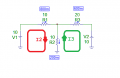Last edited:

#### dcbingaman

Joined Jun 30, 2021
855
Hello again,

You just wrote:
"But I2 IS the current flowing in V1 and R1. Measure the current in R1 and, guess what, you have just measured I2."

Very sorry but that is the same mistake that other people make too. They see the current loop arrow and follow it around then assume that it is the current in the wire just because they are used to seeing real current arrows that show the direction of the real current. I2 does not show anything real it is completely fictitious and can not be measured as i will show in this next diagram. The previous diagram had shown this too but the presence of the zero current in R2 may trip people up so i made the same circuit but with an imbalance so we would get three real currents to work with.
Notice that the currents shown with the small blue arrows next to the resistors are all real currents and every one of them can be measured.

Now looking at that new diagram, we see the real current in iR1 is:
iR1=iR2+iR3
and since iR2 is 200ma and iR3 is 600ma the current though R1 is 800ma (as shown).
So the current through R1 can not be I2 because it's too large. The current though R2 is only 200ma. Also, the current through R2 can not go in two directions at the same time, except in pure theory.

So if we measure iR1 we get 800ma. It's impossible for that current to be the same as I2 no matter where you look.
Also, you can see the small blue current arrows that indicate the direction of current in each resistor. You can see that the blue current arrow for R3 points to the right, meaning its current must come from the left. The current obviously comes from the current through R1, so again iR1 can not be the same as I2.
A simpler view maybe is that the current through R1 is shared by the the two resistors R2 and R3 yet I2 does not flow through R3 at all.
You are certainly not a stupid man so i know you will see this if you look a little more carefully.

But i didnt just make this up it's a known fact about mesh currents. You will still see people on the web claiming to 'measure' a mesh current with a multimeter. They do the same as above where they would measure the current in R1 and claim that is the mesh current I2. They say, "Put this lead here, and this other lead there, and measure the mesh current". But it is impossible and that's a known fact and that should be found on the web also but this time the statements will be trueIf this isnt clear now im not sure what else to sayexcept look at Wikipedia where they say the same thing that mesh currents are not the same.

Here's the new circuit to consider...

View attachment 275002
ok, here is the steps to a solution:

Define mesh equations: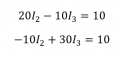Simplify: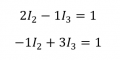Add 2 times last equation to top equation to eliminate I2 and then solve for I3: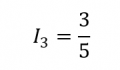Solving for I2: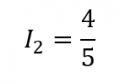The voltage across R1 is: R1*I2=10*4/5=8V
The voltage across R2 is: R2*(I2-I3)=(4/5-3/5)*10=2V
The voltage across R3 is: R3*I3=20*3/5=12V

No issues becasue:
The voltage around loop 2 is:
-10+8+2=0
The voltage around loop 3 is:
-10+-2+12=0

Verified.

But I2 is 800mA and that is going through R1 and must be coming from V1.
And I3 is 600mA and is going through R3
The current through R2 is 800mA-600mA which is 200mA and thus a 2 volt drop is correct.

The loop currents are what I expect. Not sure what you are trying to say?

The voltage across R3 is not to surprising being when you look at the outer loop the the two voltage sources are in series allowing for up to 20V in the circuit.Yep 800mA is the current through R1 and that happens to be the I2 current that I calculated so it is the I2 current which is 800mA?

So WBahn is correct: The calculated I2 current is 800mA and that is the current going through R1.

Also because both currents have positive values, the assumed direction of current flows (clockwise) is correct.

Last edited:

#### WBahn

Joined Mar 31, 2012
28,185
I agree with you, though most textbooks I have read tend to write the above equations even more abbreviated as in:

View attachment 274998

or even more concise and drop the i1 and i2 (assume them):

View attachment 274999

Not that it is correct, but it happens a lot.
Yep. And it drives me crazy.

I firmly believe that the root cause of this is poor math teachers. Now, having said that in blunt terms, let me say that it is an unintentional progression of sloppiness that is tied to most educators, at all levels, having little or not experience outside of the classroom. When students are first introduced to "story problems" that should involve the proper tracking of units, they are treated as just problems where students have to figure out how to pull out the right numbers and crank some math. The problems are initially so contrived that either they don't have any meaningful or the units would work out without any effort even if they were tracked. The units are almost always completely ignored on the belief is that introducing the issue of units in physical measures at the same time as introducing the process of extracting data and relationships from textual problems is too much. The fact that it's not and that introducing them consistently and rigorously at this early stage would let students see the value in tracking them (as they start catching their many mistakes) and get them in the habit of doing so. But the teachers putting together the curriculum and lessons only want to deal with the manipulation of numbers and often see the physical meaning and interpretation of the problems as nothing more than distractions -- and I've seen several texts that effectively state this and tell students not to be distracted by the words, focus on just the numbers and the operations implied by key words in the problem statement.

So students get set up by their math teachers to be sloppy with units. When (and if) they take a (decent) chemistry or physics class, they are usually introduced to the proper treatment of units. In chemistry classes, unfortunately, this is often limited to using "the factor-label method" to perform conversions. Physics courses are usually better about insisting that units be tracked, but even here that is not always the case and some only insist on the units being present on the answer, which completely defeats the purpose.

When you get to most engineering fields it becomes truly abhorrent because most texts will go to some lengths in the first chapter to stress how important units are, and then proceed to work every example in the book without properly tracking units and just being sure to tack what is believed to be the desired units onto the final answer. The reason is pretty simple -- most (not all, but most) engineering professors and textbook authors have little, if any, industrial work experience. For nearly their entire career, a units mistake merely caused an answer to be wrong and was therefore something that was corrected in the lesson notes or the textbook errata.

As an example, I provided feedback on a linear systems and transform methods text that is widely used and the response I got from the author was extremely positive as I pointed out errors ranging from minor typos to conceptual errors in explanations. Overall, it was a pretty decent book. But, like all the others, only paid lip service to the proper tracking of units. Then there was an example problem that had a wrong answer and it was exactly because units were not tracked and an error was made that would have affected the units in the work making them nonsensical, but the expected units were simply tacked on the end. When I sent this to the author, and used it as an example of why the text needed to at least model the proper tracking of units throughout the worked examples, I got the cold shoulder. Not only did I not get the usual acknowledgement for that suggestion, but my subsequent suggestions went ignored as well. In the next edition of the book that mistake was fixed (in terms of numbers) but the units were still not tracked in the work.

So it is little surprise that most working engineers are very poorly educated when it comes to the proper use and tracking of units. It's also not too surprising to see them get ultra defensive when this is pointed out to them. It's human nature.

There's virtually nothing we can do about K-12 education in this regard, but I strongly believe that organizations like ABET should incorporate it into their standards. But, having worked with ABET a few times, I don't see that happening, either.

#### WBahn

Joined Mar 31, 2012
28,185
View attachment 275002

You just wrote:
"But I2 IS the current flowing in V1 and R1. Measure the current in R1 and, guess what, you have just measured I2."

Very sorry but that is the same mistake that other people make too. They see the current loop arrow and follow it around then assume that it is the current in the wire just because they are used to seeing real current arrows that show the direction of the real current. I2 does not show anything real it is completely fictitious and can not be measured as i will show in this next diagram. The previous diagram had shown this too but the presence of the zero current in R2 may trip people up so i made the same circuit but with an imbalance so we would get three real currents to work with.
Notice that the currents shown with the small blue arrows next to the resistors are all real currents and every one of them can be measured.

Now looking at that new diagram, we see the real current in iR1 is:
iR1=iR2+iR3
and since iR2 is 200ma and iR3 is 600ma the current though R1 is 800ma (as shown).
So, if I am extracting your claims correctly, you are saying that IR1 is 800 mA, IR2 is 200 mA, and IR3 is 600 mA.

Okay. No problem.

So the current through R1 can not be I2 because it's too large.
Then what, pray tell, do YOU claim the value of I2 is? It's not enough to just assert that it can't be that large. What is it? How did you arrive at that smaller value?

Let's see what mesh analysis claims:

Mesh 1: I2*(R1+R2) - I3*(R2) = V1
Mesh 2: -I2*(R2) + I3*(R2+R3) = V2

I2*(R1+R2)(R2+R3) - I3*(R2)(R2+R3) = V1*(R2+R3)
-I2*(R2)(R2) + I3*(R2)(R2+R3) = V2*(R2)

I2*(R1*R2+R1*R3+R2*R3) = V1*(R2+R3) + V2*(R2)

I2 = [V1*(R2+R3) + V2*(R2)] / (R1*R2+R1*R3+R2*R3)

I2 = [(10 V)*(10 Ω + 20 Ω) + (10 V)*(10 Ω)] / ((10 Ω)*(10 Ω)+(10 Ω)*(20 Ω)+(10 Ω)*(20 Ω))

I2 = (300 VΩ + 100 VΩ) / (100 Ω² + 200 Ω² + 200 Ω²)

I2 = (400 VΩ) / (500 Ω²) = 0.8 A = 800 mA

What was that you were saying about there being no way for I2 to be 800 mA???

Your entire argument rests on the claim that I2 has to be smaller than the 800 mA flowing through iR2.

It's not.

What does mesh analysis have to say about the value of I3?

I3 = [V2 + (I2*R2)] / (R2 + R3)

I3 = [10 V + (800 mA)(10 Ω)] / (10 Ω + 20 Ω)

I3 = (10 V + 8 V) / 30 Ω = 18 V / 30 Ω = 0.6 A = 600 mA

The current though R2 is only 200ma.
Agreed. So?

Mesh current analysis says that

iR2 = I2 - I3 = 800 mA - 600 mA = 200 mA

Surprise, surprise.

Also, the current through R2 can not go in two directions at the same time, except in pure theory.
No one is saying that it is. The current through R2, in the direction indicated by the arrow near it, is neither I2 nor I3, it is (I2-I3).

So if we measure iR1 we get 800ma. It's impossible for that current to be the same as I2 no matter where you look.
Really?

Seems like if you measure iR1 and get 800 mA, you just got the same current as I2.

Similarly, if you measure iR3 and get 600 mA, you just measured I3, which is 600 mA.

Please show a circuit in which the branch current in any branch that only has one mesh current going through it is not equal to that mesh current. This MUST be true as long as charge is conserved. It is even true in non-linear circuits, the only difference is that you can't separate the mesh currents and superimpose their individual effects.

Last edited:

#### MrAl

Joined Jun 17, 2014
9,781
View attachment 275002

So, if I am extracting your claims correctly, you are saying that IR1 is 800 mA, IR2 is 200 mA, and IR3 is 600 mA.

Okay. No problem.

Then what, pray tell, do YOU claim the value of I2 is? It's not enough to just assert that it can't be that large. What is it? How did you arrive at that smaller value?

Let's see what mesh analysis claims:

Mesh 1: I2*(R1+R2) - I3*(R2) = V1
Mesh 2: -I2*(R2) + I3*(R2+R3) = V2

I2*(R1+R2)(R2+R3) - I3*(R2)(R2+R3) = V1*(R2+R3)
-I2*(R2)(R2) + I3*(R2)(R2+R3) = V2*(R2)

I2*(R1*R2+R1*R3+R2*R3) = V1*(R2+R3) + V2*(R2)

I2 = [V1*(R2+R3) + V2*(R2)] / (R1*R2+R1*R3+R2*R3)

I2 = [(10 V)*(10 Ω + 20 Ω) + (10 V)*(10 Ω)] / ((10 Ω)*(10 Ω)+(10 Ω)*(20 Ω)+(10 Ω)*(20 Ω))

I2 = (300 VΩ + 100 VΩ) / (100 Ω² + 200 Ω² + 200 Ω²)

I2 = (400 VΩ) / (500 Ω²) = 0.8 A = 800 mA

What was that you were saying about there being no way for I2 to be 800 mA???

Your entire argument rests on the claim that I2 has to be smaller than the 800 mA flowing through iR2.

It's not.

What does mesh analysis have to say about the value of I3?

I3 = [V2 + (I2*R2)] / (R2 + R3)

I3 = [10 V + (800 mA)(10 Ω)] / (10 Ω + 20 Ω)

I3 = (10 V + 8 V) / 30 Ω = 18 V / 30 Ω = 0.6 A = 600 mA

Agreed. So?

Mesh current analysis says that

iR2 = I2 - I3 = 800 mA - 600 mA = 200 mA

Surprise, surprise.

No one is saying that it is. The current through R2, in the direction indicated by the arrow near it, is neither I2 nor I3, it is (I2-I3).

Really?

Seems like if you measure iR1 and get 800 mA, you just got the same current as I2.

Similarly, if you measure iR3 and get 600 mA, you just measured I3, which is 600 mA.

Please show a circuit in which the branch current in any branch that only has one mesh current going through it is not equal to that mesh current. This MUST be true as long as charge is conserved. It is even true in non-linear circuits, the only difference is that you can't separate the mesh currents and superimpose their individual effects.
Hello again,

Hey thanks for sticking with this.

Ok why do you have to call it a 'mesh' current then. Why cant you just call it a 'current'.
My argument is that if you show a current path with an arrow then that current has to be present in all parts of that path. It cant be present in R2 because if it was we would have 800ma though R1 splitting into 800ma and 600ma which is 1400ma. So saying that I2 'flows' through R2 can not be right. The sum of the currents entering a node has to be the same as the sum leaving the nodes. If 800ma is going through R1 and R2 and 600ma through R3 then the sum would be 800ma-800ma+600ma at the central node. So my calling it a 'mesh' current we pretend it's real, that's all. The math works because of the principle of superposition. But in superposition, once we combine quantities we can no longer distinguish what the two quantities were. So if i say we have 200ma flowing through a resistor, we wouldnt be able to tell anything real other than there is 200ma flowing through that resistor. It could have been 800ma one way and 600ma the other way, or 900ma one way and 700ma the other way, or any combination that works out to 200ma. But looking inside the physical resistor, we are not going to see two different currents flowing we are going to see everything going in one direction, and it just so happens that the current and the direction is going to coincide with any of the two 'imaginary' currents that we suppose to be BOTH flowing through the same resistor, and in this case in opposite directions.
OK, so how can we have two real currents flowing through a resistor in opposite directions. We cant. That would also produce two different magnetic fields when we could only really have one field.

Now we can 'say' that there is a current of 800ma through R2, but that will never happen in that circuit.
What we might be able to do is pump 800ma through R2 for 1ms and 600ma back the other way for the next 1ms, then keep alternating and take the average. But the average of +800 and -600 is not +200 it is +100, so that doesnt work at all. It has to be 800ma for a 1ms period and -600ma for the SAME 1ms period which doesnt make sense.

What you might want to do to understand my point is to break that loop arrow I2 into a bunch of shorter arrows that follow the original loop arrow around, then ask what is the real current in each smaller segment. In the top R1 you see 800ma with the smaller segments close to R1, but as you get to R2 you see 200ma in the smaller segments near R2, so this current that follows a continuous path changes from 800ma to 200ma, but we still call it I2. I2 cant be BOTH 800ma and 200ma. We "assign" it the value of 800ma for the purpose of a calculation, that's all. It appears to work because of superposition, but there is no REAL addition or subtraction going on inside R2.

This issue is a little different than what we usually run into i think that's the problem. If i hand someone a 9v battery and they measure 9v, then hand them a second battery and they measure 9v with that one, then they give them back to me and i mix them up with their back turned then turn them around and ask which battery did i hand to them first, they wont be able to tell me even after they measure the two batteries because there is no way to tell them apart physically, but in reality they are two physically different, separate batteries.

I will just point out one more thing. If we have to qualify the mesh currents with the word "component" then why would we have to do that if they are just the same real currents as we find in a single series circuit. We do that to try to explain the difference, and there is a difference that's why we have to do that.

I was going to say that there are some circuits that mesh analysis does not work in without changing the topology, but this may not be that uncommon because we have to do that with nodal also sometimes. I might look into that at some point but i think we are starting to spend too much time on this because if mesh analysis works then it is still a good tool to have, regardless how you interpret the mesh 'currents'.